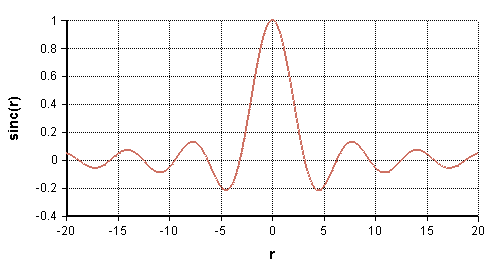# Integrate

## Integrate(y, x, i)

Returns the result of applying the trapezoidal rule of integration to the set of points (xi, yi).

Integrate computes the cumulative integral across «i», returning a value with the same number of dimensions as «x» and «y». Compare Integrate to Area.

When either «x» or «y» is an index, the index parameter «i» may be safely omitted.

Array functions

## Examples

Function Sinc(x) := If x = 0 Then 1 Else Sin(Degrees(x))/x
Index r := Sequence(-20, 20, 0.01)
Sinc(r) →.

The integral of this function is given by:

Integrate(Sinc(r), r, r) →Note that because the index r was defined from -20 to 20, Integrate treats all values for x < -20 as being zero.

## Handling of Null values

The «x» and «y» arrays must contain numbers, but may also contain Null values. Any point having «x» = Null is entirely ignored and has no impact on the result. When a point has a numeric «x» but a Null-valued «y», this is treated as a gap in the function, and the trapezoid extending from the previous «x» to the next «x» is not counted in the integration.

Suppose, for example, that:

Index x := 1..5
Variable y := If x = 3 then Null else x

In this example, the curve denoted by (x, y) has a gap at x = 3. Integrate treats this as if it were the function shown here:

y →Integrate(y, x) →In this case, the area to the left of x = 1 is 0, the area to the left of x = 2, x = 3 or x = 4 is 1.5, and the area to the left of x = 5 is 1.5 + 4.5 = 6.

## Functions with Discontinuities

To represent a function with a discontinuity, you must include the same value in «x» twice, being the value where the discontinuity occurs. The first occurrence of that value provides the value to the immediate left of the «x» point, while the last occurrence of that particular «x» value is the value to the immediate right.

## Ordering of points

Starting with Analytica 4.2, Integrate treats the (x, y) points as a set of points, in which the ordering of points doesn't impact the value. Thus, the points do not need to be sorted to be increasing in «x», and the result is not impacted by shuffling the order of the points. There is, however, one exception to this ordering invariance -- the case in which the same «x» value occur multiple times. Multiple occurrences of the same «x» encodes a discontinuity in the function (see previous section). In this case, the first occurrence of a given value is always used as the value to the immediate left, and the final occurrence is always used as the value to the immediate right.